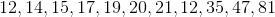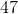# Algebra 1 : How to find mode

## Example Questions

### Example Question #121 : How To Find Mode

Find the mode of the following set: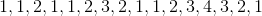Explanation:

To find the mode of any set, find the number which occurs the greatest number of times in the set.  The best answer is:### Example Question #122 : How To Find Mode

Find the mode of the following set: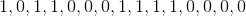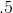Explanation:

To find the mode of any set, find the number which occurs the greatest number of times in the set.  The best answer is:### Example Question #123 : How To Find Mode

Find the mode of the following set: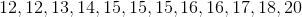Explanation:

To find the mode of any set, find the number which occurs the greatest number of times in the set.  The best answer is:### Example Question #124 : How To Find Mode

Find the mode of the following set: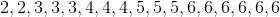Explanation:

To find the mode of any set, find the number which occurs the greatest number of times in the set.  The best answer is:### Example Question #125 : How To Find Mode

To find the mode of any set, find the number which occurs the greatest number of times in the set.  The best answer is: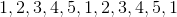Explanation:

To find the mode of any set, find the number which occurs the greatest number of times in the set.  The best answer is:### Example Question #121 : How To Find Mode

Find the mode: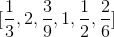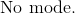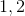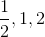Explanation:

The mode is the highest frequency of number or numbers in a dataset.

There are fractions that are not fully reduced.  Simplify those fractions and rewrite the dataset.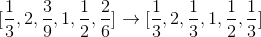We can now see thathas the highest frequency of occurrence.

The answer is:### Example Question #122 : How To Find Mode

Find the mode of the following set: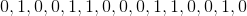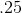Explanation:

To find the mode of any set, find the number which occurs the greatest number of times in the set.  The best answer is:### Example Question #123 : How To Find Mode

Find the mode of the following set: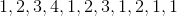Explanation:

To find the mode of any set, find the number which occurs the greatest number of times in the set.  The best answer is:### Example Question #129 : How To Find Mode

Find the mode of the following set: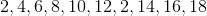Explanation:

To find the mode of any set, find the number which occurs the greatest number of times in the set.  The best answer is:### Example Question #124 : How To Find Mode

Find the mode of the following set: